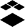## Using Formula Questions to Convert Hex to DecimalCommunity Novice

Hey All,

I'd like to think myself pretty familiar with the new style of Canvas quizzes; however, I want to see if I can further expand my knowledge.

This semester, I am teaching a digital electronics class. Part of the class is understanding number systems, such as base-2 (binary) and base-16 (hex).

My question is hopefully simple to solve; or perhaps a solution does not currently exist:

Can I write a formula question that converts hex to base 10, using variables?

Doing so with binary was straight-forward:

• Set variables `a` through `e` for a 5bit number.
• Set Min/Max to be 0/1 respectively
• Set formula definition: (a*2^4) + (b*2^3)... and so on.
• Generate solutions

With Hex, digits go from 0 to F (which represents 15); not-so-possible with variables that can only have numeric Min/Max values.

Labels (1)
• ### Canvas

1 SolutionInstructure Alumni

I looked into this for you and was unable to find a way to achieve this using New Quizzes.

This would make a great feature request.